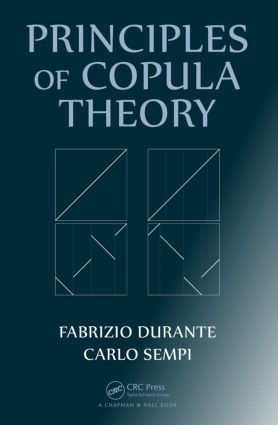# Principles of Copula Theory

## 1st Edition

Chapman and Hall/CRC

332 pages | 19 B/W Illus.

##### Purchasing Options:\$ = USD
Hardback: 9781439884423
pub: 2015-07-07
SAVE ~\$22.00
\$110.00
\$88.00
x
eBook (VitalSource) : 9780429066399
pub: 2015-07-01
from \$28.98

FREE Standard Shipping!

### Description

Principles of Copula Theory explores the state of the art on copulas and provides you with the foundation to use copulas in a variety of applications. Throughout the book, historical remarks and further readings highlight active research in the field, including new results, streamlined presentations, and new proofs of old results.

After covering the essentials of copula theory, the book addresses the issue of modeling dependence among components of a random vector using copulas. It then presents copulas from the point of view of measure theory, compares methods for the approximation of copulas, and discusses the Markov product for 2-copulas. The authors also examine selected families of copulas that possess appealing features from both theoretical and applied viewpoints. The book concludes with in-depth discussions on two generalizations of copulas: quasi- and semi-copulas.

Although copulas are not the solution to all stochastic problems, they are an indispensable tool for understanding several problems about stochastic dependence. This book gives you the solid and formal mathematical background to apply copulas to a range of mathematical areas, such as probability, real analysis, measure theory, and algebraic structures.

### Reviews

"This book presents an overview of the theory of copulas. The research on copulas and its applications has grown very rapidly from Sklar's seminal paper in 1959. Recent applications in finance have boosted new research, and several books have been written on this subject during the last two decades.

… In this book, the authors' focus is on the mathematical foundations of the theory of copulas. In fact, the first chapters are devoted to put the theory of copulas into the frame of measure theory. Moreover, the theory is presented for general d-copulas (d > 2), whenever possible. Pointers to applications are given in special paragraphs named \Further readings"…. The presentation is always clear and the mathematical language is precise. The book can be used as a reference book for mathematicians and statisticians interested in the theory of copulas, and especially in the mathematical foundations of that theory. Moreover, the book can also be useful to those researchers in applied fields who need a strong reference manual on the mathematical aspects of the theory of copulas."

—Fabio Rapallo (Alessandria) in Zentralblatt Mathematik, April 2018

"This book represents a rigourous introduction to the theory of copula models, the biggest and the most thorough yet at that. The level of detail and rigour targets mathematicians working in probability theory. The exposition starts with an overview of the history of the subject followed by eight chapters laying out the theory of Copula models. The idea of the book was to present modern theoretical foundations for Copula models now that the field has seen over 50 years of research that has greatly accelerated recently with the development of applications in Finance, Operations Research, Statistics and Biostatistics. … For a mathematically-oriented researcher in Statistics, Biostatistics and Applied Probability, and further in the specific applied fields of science, the book will serve as a reference for theoretical ideas potentially inspiring applied theory development."

— Alex Tsodikov, University of Michigan, in International Statistical Review, December 2017

Copulas: Basic Definitions and Properties

Notations

Preliminaries on random variables and distribution functions

Definition and first examples

Characterization in terms of properties of d.f.s

Continuity and absolutely continuity

The derivatives of a copula

The space of copulas

Graphical representations

Copulas and Stochastic Dependence

Construction of multivariate stochastic models via copulas

Sklar’s theorem

Proofs of Sklar’s theorem

Copulas and risk-invariant property

Characterization of basic dependence structures via copulas

Copulas and order statistics

Copulas and Measures

Copulas and d-fold stochastic measures

Absolutely continuous and singular copulas

Copulas with fractal support

Copulas, conditional expectation, and Markov kernel

Copulas and measure-preserving transformations

Shuffles of a copula

Sparse copulas

Ordinal sums

The Kendall distribution function

Copulas and Approximation

Uniform approximations of copulas

Application to weak convergence of multivariate d.f.s

Markov kernel representation and related distances

Copulas and Markov operators

Convergence in the sense of Markov operators

The Markov Product of Copulas

The Markov product

Invertible and extremal elements in C2

Idempotent copulas, Markov operators, and conditional expectations

The Markov product and Markov processes

A generalization of the Markov product

A Compendium of Families of Copulas

What is a family of copulas?

Fréchet copulas

EFGM copulas

Marshall-Olkin copulas

Archimedean copulas

Extreme-value copulas

Elliptical copulas

Invariant copulas under truncation

Generalizations of Copulas: Quasi-Copulas

Definition and first properties

Characterizations of quasi-copulas

The space of quasi-copulas and its lattice structure

Mass distribution associated with a quasi-copula

Generalizations of Copulas: Semi-Copulas

Definition and basic properties

Bivariate semi-copulas, triangular norms, and fuzzy logic

Relationships among capacities and semi-copulas

Transforms of semi-copulas

Semi-copulas and level curves

Multivariate aging notions of NBU and IFR

Bibliography

Index

### About the Authors

Fabrizio Durante is a professor in the Faculty of Economics and Management at the Free University of Bozen–Bolzano. He is an associate editor of Computational Statistics & Data Analysis and Dependence Modeling. His research focuses on multivariate dependence models with copulas, reliability theory and survival analysis, and quantitative risk management. He earned a PhD in mathematics from the University of Lecce and habilitation in mathematics from the Johannes Kepler University Linz.

Carlo Sempi is a professor in the Department of Mathematics and Physics at the University of Salento. He has published nearly 100 articles in many journals. His research interests include copulas, quasi-copulas, semi-copulas, weak convergence, metric spaces, and normed spaces. He earned a PhD in applied mathematics from the University of Waterloo.

### Subject Categories

##### BISAC Subject Codes/Headings:
MAT000000
MATHEMATICS / General
MAT029000
MATHEMATICS / Probability & Statistics / General
MAT029010
MATHEMATICS / Probability & Statistics / Bayesian Analysis